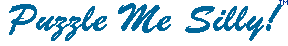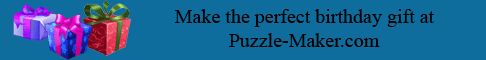Cliches 2--When life is hard ``` 1. Y D E M W X S X F Y B J Y F K W Y X Q K W Q M A K D E M Y F K W B S E F S M E , X F M B Y T M X K T K B C Y X F B S P M M X ``` ``` 2. T S E X S P X F M T S D B X K Y B E C M F K H M Y B N Y P M K I M S B M E C M Q D Y N W S D I E M N H M E . ``` ``` 3. C F M B L S W L Y H M E V S D N M T S B E , T K J M N M T S B K W M ``` ``` 4. N Y P M Y E K E M I Y M E S P W Y E K O O S Y B X T M B X E , P S N N S C M W Q V W M K X F ``` ``` 5. N Y P M Y E B S X F K I W , Y X S B N V B M M W E E S T M O S E Y X Y H M X F Y B J Y B L ``` ``` 6. Y X Y E K L S S W M U O M I Y M B A M , Y X T K J M E T M E X I S B L M I ``` ```  ``` Copyright © 2012 Variety Games Inc.